# Analyzing motion in connected rigid bodies (Part - 1) Civil Engineering (CE) Notes | EduRev

## Civil Engineering (CE) : Analyzing motion in connected rigid bodies (Part - 1) Civil Engineering (CE) Notes | EduRev

The document Analyzing motion in connected rigid bodies (Part - 1) Civil Engineering (CE) Notes | EduRev is a part of the Civil Engineering (CE) Course Introduction to Dynamics and Vibrations- Notes, Videos, MCQs.
All you need of Civil Engineering (CE) at this link: Civil Engineering (CE)

6.3 Analyzing motion in connected rigid bodies

The formulas in 6.2.3 are used to analyze motion in machines.   A typical problem is illustrated in the figure.  An actuator moves point B on the car jack shown in the figure horizontally with constant velocity V.  What are the velocity and acceleration of the platform (CF)?

You could probably solve this rather simple example with elementary trig, but we need a more systematic method for general problems, especially to analyze 3D motion.   Here’s the general procedure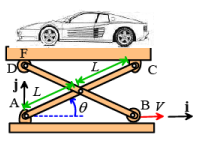1. Define variables to denote the unknown angular velocities and angular accelerations of each rigid body in the system
2. Write down all the known velocities in the system
3. Use the rigid body formulas vB −vA = ω ×(rB − rA) to write down equations relating velocities of the connections, joints, or contacts on each rigid body
4. Write down constraint equations relating velocities of the two connected rigid bodies at each connection, joint, or contact
5. Solve the equations for unknown velocities of connections, and the angular velocities of the rigid bodies.
6. Finally, once the velocities are known,  write down equations for the accelerations of pairs of joints/contacts/connections on each rigid body  aB − aA = α ×(rB − rA) + ω×(vB − vA)
7. Write down constraints equations for accelerations at connected points
8. Solve the equations in 6,7 for unknown accelerations and angular accelerations.

This all sounds terribly complicated, so let’s solve a few examples to show how it works in practice.

Example 1: In the figure shown the link AB rotates counterclockwise with constant angular speed 4 rad/s.     Point C on member BC is constrained to move horizontally.  Calculate the velocity and acceleration of point C.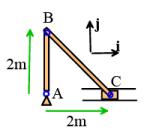Calculating the velocity:

• We know A is stationary, and are given the angular velocity of AB.  We can use the rigid body formula to find the velocity of B:

VB - VAzABk x (rB - rA) = 4k x 2j

⇒vB = -8i

• We don’t know the angular velocity of BC, so we introduce ωzBC as an unknown, and use the rigid body formula for member BC to write down an equation for the velocity of C

vc - vB = ωzBck x (rc - rB) = ωzBck x (2i - 2j) = 2ωBCi + 2ωBCj

⇒ v= -8i + 2ωBC1 + 2ωBCj

• We know that C can only move horizontally. This means that its j component of velocity must be zero.   This shows that

ωBC =0, vC = −8i

Calculating the acceleration:

• We know A is stationary, and are given the angular velocity and angular acceleration of AB. We can use the rigid body formula to find the acceleration of B:

aB - aA = αzABk x (rB - rA) - ω2zAB (rB - rA) = -32j

⇒ aB = -32 j

• We don’t know the angular acceleration of BC, so we introduce αzBC as an unknown and use the rigid body formula for member BC to write down an equation for the acceleration of C

aC - aB = αzBCk x (rC - rB ) - ω2zAB (rC - rB ) = αzBCk x (2i - 2 j) - 0

⇒ aC = -32j + 2αBCi + 2αzBCj

• Point C can only move horizontally, so it can’t have any vertical acceleration.  This means that the j component of acceleration is zero:

zBC - 32 - 0 ⇒αzBC - 16

⇒aC = 32i

Example 2: For a more complicated example, we can solve the car jack problem posed at the start of this section. An actuator moves point B on the car jack shown in the figure horizontally with constant velocity V. What are the velocity and acceleration of the platform (CF)?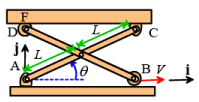The system contains 3 rigid bodies (AC, BD, CF3).  We don’t know the angular velocities or accelerations of any of them, so we denote them by unknowns ωzAC

ω zBD ωzCFzAC, αzBD αzCF

Calculating the velocity:

• We start at point(s) with known velocity: A is stationary, and the velocity of B is given:

vA = 0 vB = Vi

• Point E lies on both member AC and on member BD. We use the rigid body formulas to write down an equation for the velocity of E on each member (notice we use the 2D equations):

vE - vA = ωzACk x (rE - rA)

vE - vB = ωzBDk x (rE - rB)

• The two members AC and BD are pinned together at E and so must have the same velocity.  We can eliminate vE and write out the position vectors in i,j components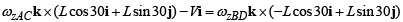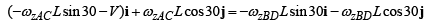The i,j components give two equations for ωzAC zBD

zACLsinθ - V = -ωzBDLsinθ

ωzAcLcosθ = -ωzBDLcosθ

⇒ωzAC 2L sinθ cos θ-V cosθ = 0

⇒ωzAC =-V/(2L sinθ)   ωzBD = V/(2L sinθ)

• We can now use the rigid body formulas for members AC and BD to find the velocities of C and D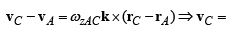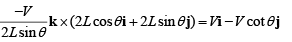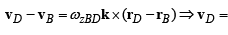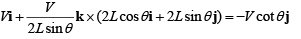• We can use the rigid body formula for CF to relate the velocities of C and F

vF - VC - ωzCFk x (rF - rC )
VF= Vi - V cot θj - ωzCF 2Lcosθ j

• Point D on CD and point F on CF must have the same vertical velocity (the roller at D allows their horizontal velocities to differ). This can be expressed as

yF ' j = vD ·j ⇒-Vcotθ - ωZCF2Lcosθ = -Vcotθ

⇒ωZCF =0

• All points on CF therefore have the same velocity (equal to the velocity of C)

vCF = Vi − V cotθj

Calculating the acceleration.

• We can now calculate the accelerations.  We start at a known point: Points A and B have zero acceleration.
• We can use the rigid body formula to calculate the acceleration of E on each of AC and BD: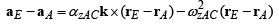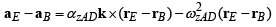• The two members are connected at E and so must have the same acceleration there.  This shows that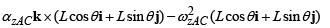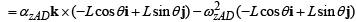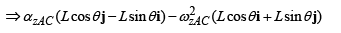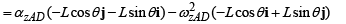• The i,j components give two equations for the unknown angular accelerations: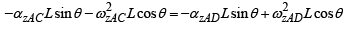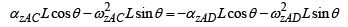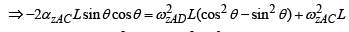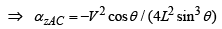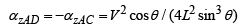• We can use the rigid body acceleration formulas to calculate the velocities of D and C: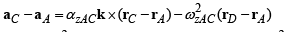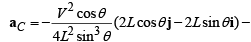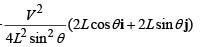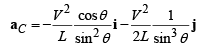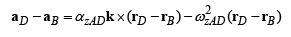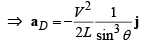• We can use the rigid body formula to relate the accelerations of C and F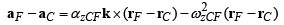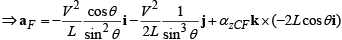• Finally, we know that D and F must have the same vertical acceleration (so they remain in contact).  Their horizontal accelerations may differ, because of the roller attached to D.   This gives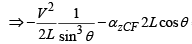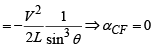• Since CF has zero angular velocity and angular acceleration, all points on CF have the same acceleration (which must equal that of point C).   Therefore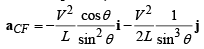Offer running on EduRev: Apply code STAYHOME200 to get INR 200 off on our premium plan EduRev Infinity!

## Introduction to Dynamics and Vibrations- Notes, Videos, MCQs

20 videos|53 docs

,

,

,

,

,

,

,

,

,

,

,

,

,

,

,

,

,

,

,

,

,

;